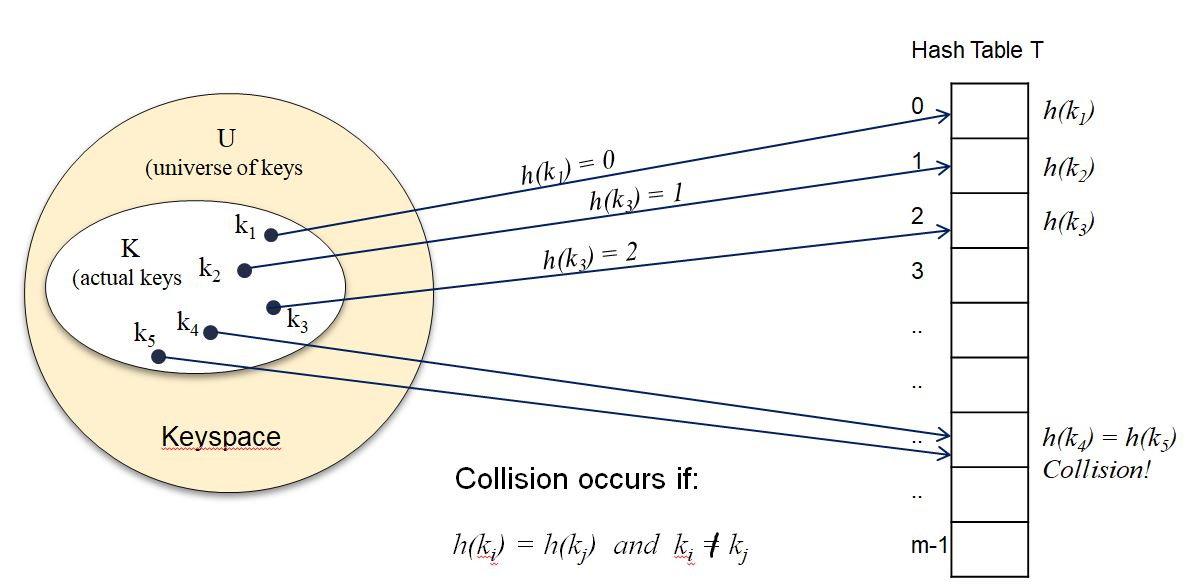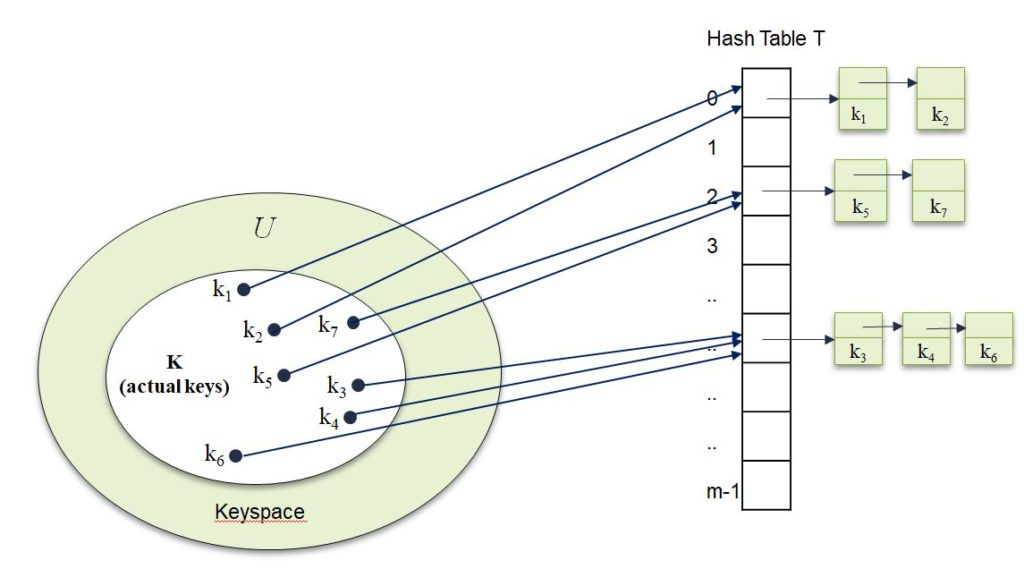# Hash Tables – Hashing With Chaining

Hashing With Chaining. In the discussion of direct addressing, we see that for a fairly small-size universe U, we can use a direct-address table. But when the universe is large then it would not be practical to create a direct-address table T of size |U|.

Also, the set of K of the actual keys stored in T may be very small relative to the size if U that space may be wasted.

We would cover the following:

##### 1. How Hashing Works

With direct addressing, the element with the  key k is placed in slot k. With hashing, this element is stored in the slot h(k). This means that we use a hash function h(k) to compute the slot using the key. The hash function h maps the universe U into slots in the table T[0, 1, 2, . . .  m-1]

h: U → {0, 1, 2, . . . , m-1}

where the size of the hash table m is much less than the size of the universe U.

Here we say that  the element with key k hashes to slot h(k) and that h(k) is the has value of the key k. The hash function is used to reduce the range of the array indices to the size of the hash table. This is illustrate in Figure 1.0.Figure 1.1: The hash function h maps the keys from the universe to the slots in the hash table

Collision occurs if two keys map to the same slot in the hash table. One method of resolving collision is by chaining as we would discuss next.

##### 2. Resolving Collision by Chaining

One method of handling collision in a hash table is by chaining. In chaining,  we use a linked list to manage collision. Elements that hash to the same slot are placed in a linked list and the slot in the hash table would contain a pointer to the head of this hash table. This is shown in Figure 1.3Figure 1.3: Each slot in the hash table T[j] contains a linked list of all the keys that hash to that slot. In the figure the linked list is singly-linked but it can also be doubleThe dictionary operations on a hash table where collision is resolve by chaining is given below

```SEARCH (T, k)
search for element with key k in the list T[h(k)]

INSERT (T, x)
insert x at the head of hte list T[h(x.key)]

DELETE (T, x)
delete x from the list T[h(x.key)]
```

The worst-case running time for insertion is O(n)

##### 3. Analysis of Hashing With Chaining

We will try to determine how long it takes to search for an element with a given key k.

Given a hash table T that have m slot and stores n elements,  a value known as the load factor α can be defined and is given as α = n/m

This means the average number of  elements  stored in a chain. The value of α could be less than, equal to or greater than 1. In the worst case , all the keys hashes to the same slot and all we would have is one large linked list of length n. This would give a worst-case search time of Θ(n) plus the time needed to compute the hash functions.

In the average case, the performance would depend on how well the hash function h distributes the keys among the m slots of the hash table.

##### 4. Simple Uniform Hashing

Let’s assume that any given element has equal likelihood of being hashed into any of the m slots of the hash table independent of where any other element is hashed to. This assumption is known as Simple Uniform Hashing.

For i = 0, 1, 2, . . . , m-1, lets denote the length of the list T[j] by nj such that

n = n0  + n1 + . . .  + nm-1

The expected length of the chain nj is E[nj] = n/m = α

Watch the Video Lesson

##### Check these Data Structure and Algorithms Tutorials#### kindsonthegenius

Kindson Munonye is currently completing his doctoral program in Software Engineering in Budapest University of Technology and Economics

View all posts by kindsonthegenius →

## 5 thoughts on “Hash Tables – Hashing With Chaining”

1.Cierra Heany says:

2.Lili Mclauglin says:
3.Jayne Whitlach says: